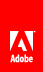# 遮罩显示对象

Flash Player 9 和更高版本，Adobe AIR 1.0 和更高版本

## 定义遮罩

```// Make the object maskSprite be a mask for the object mySprite.

```// This code assumes it's being run within a display object container
// such as a MovieClip or Sprite instance.

import flash.display.Shape;

// Draw a square and add it to the display list.
var square:Shape = new Shape();
square.graphics.lineStyle(1, 0x000000);
square.graphics.beginFill(0xff0000);
square.graphics.drawRect(0, 0, 100, 100);
square.graphics.endFill();

// Draw a circle and add it to the display list.
var circle:Sprite = new Sprite();
circle.graphics.lineStyle(1, 0x000000);
circle.graphics.beginFill(0x0000ff);
circle.graphics.drawCircle(25, 25, 25);
circle.graphics.endFill();

{
}

```// remove the mask from mySprite

## Alpha 通道遮罩

```// maskShape is a Shape instance which includes a gradient fill.
mySprite.cacheAsBitmap = true;

```// This code assumes it's being run within a display object container
// such as a MovieClip or Sprite instance.

import flash.display.Sprite;
import flash.geom.Matrix;
import flash.net.URLRequest;

var url:URLRequest = new URLRequest("http://www.helpexamples.com/flash/images/image1.jpg");

// Create a Sprite.
var oval:Sprite = new Sprite();
var colors:Array = [0x000000, 0x000000];
var alphas:Array = [1, 0];
var ratios:Array = [0, 255];
var matrix:Matrix = new Matrix();
colors,
alphas,
ratios,
matrix);
oval.graphics.drawEllipse(-100, -50, 200, 100);
oval.graphics.endFill();
// add the Sprite to the display list